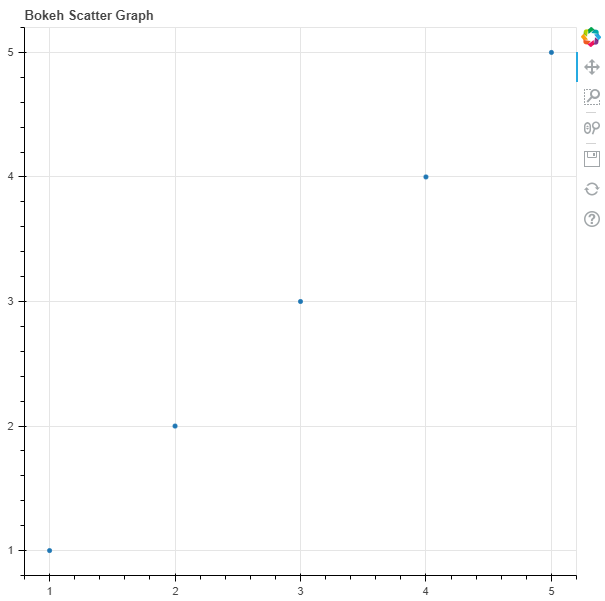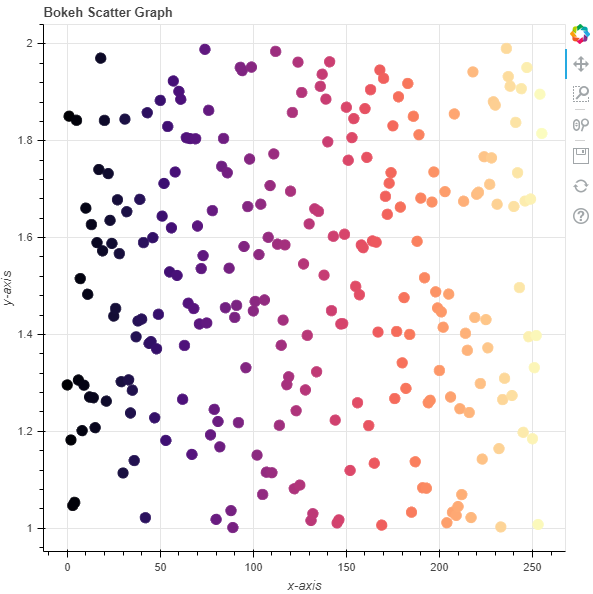# Python Bokeh – Plotting a Scatter Plot on a Graph

Bokeh is a Python interactive data visualization. It renders its plots using HTML and JavaScript. It targets modern web browsers for presentation providing elegant, concise construction of novel graphics with high-performance interactivity.

Bokeh can be used to plot a scatter plot on a graph. Plotting squares on a graph can be done using the `scatter()` method of the `plotting` module.

## plotting.figure.scatter()

Syntax : scatter(parameters)

Parameters :

• x : x-coordinates of the center of the glyphs
• y : y-coordinates of the center of the glyphs
• marker : signifies the type of the glyph

Returns : an object of class `GlyphRenderer`

Example 1 : In this example we will be using the default values for plotting the graph.

 `# importing the modules ` `from` `bokeh.plotting ``import` `figure, output_file, show ` `       `  `# file to save the model ` `output_file(``"gfg.html"``) ` `       `  `# instantiating the figure object ` `graph ``=` `figure(title ``=` `"Bokeh Scatter Graph"``) ` `     `  `# the points to be plotted ` `x ``=` `[``1``, ``2``, ``3``, ``4``, ``5``] ` `y ``=` `[``1``, ``2``, ``3``, ``4``, ``5``] ` `    `  `# plotting the graph ` `graph.scatter(x, y) ` `     `  `# displaying the model ` `show(graph) `

Output :Example 2 : In this example we will be plotting multiple scatter points with various other parameters

 `# importing the modules  ` `from` `bokeh.plotting ``import` `figure, output_file, show  ` `from` `bokeh.palettes ``import` `magma ` `import` `random ` ` `  `# file to save the model  ` `output_file(``"gfg.html"``)  ` `      `  `# instantiating the figure object  ` `graph ``=` `figure(title ``=` `"Bokeh Scatter Graph"``)  ` ` `  `# name of the x-axis  ` `graph.xaxis.axis_label ``=` `"x-axis"` `      `  `# name of the y-axis  ` `graph.yaxis.axis_label ``=` `"y-axis"` ` `  `# points to be plotted ` `x ``=` `[n ``for` `n ``in` `range``(``256``)] ` `y ``=` `[random.random() ``+` `1` `for` `n ``in` `range``(``256``)] ` `size ``=` `10` ` `  `# color value of the scatter points ` `color ``=` `magma(``256``) ` ` `  `# plotting the graph  ` `graph.scatter(x, y, ` `              ``size ``=` `size, ` `              ``color ``=` `color)  ` `      `  `# displaying the model  ` `show(graph) `

Output :Whether you're preparing for your first job interview or aiming to upskill in this ever-evolving tech landscape, GeeksforGeeks Courses are your key to success. We provide top-quality content at affordable prices, all geared towards accelerating your growth in a time-bound manner. Join the millions we've already empowered, and we're here to do the same for you. Don't miss out - check it out now!